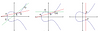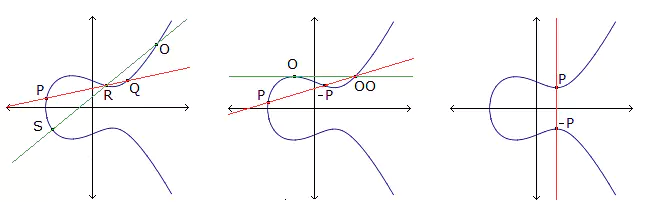# On maximal curves over finite fields of small order

### Journal article

S. Fanali, M. Giulietti, Irene Platoni

Semantic Scholar DBLP DOI
Cite

### Cite

APA
Fanali, S., Giulietti, M., & Platoni, I. (2012). On maximal curves over finite fields of small order. Adv. Math. Commun.

Chicago/Turabian
Fanali, S., M. Giulietti, and Irene Platoni. “On Maximal Curves over Finite Fields of Small Order.” Adv. Math. Commun. (2012).

MLA
Fanali, S., et al. “On Maximal Curves over Finite Fields of Small Order.” Adv. Math. Commun., 2012.

## Abstract

We show that there exists a unique maximal curve of genus $7$ over the finite field with $49$ elements, up to birational equivalence. This was the first open classification problem for maximal curves, since maximal curves over the finite fields with less than $49$ elements, as well as maximal curves over the finite field with $49$ elements with genus larger than $7$, had been previously classified. A significant role is played by some exhaustive computer searches.

Share